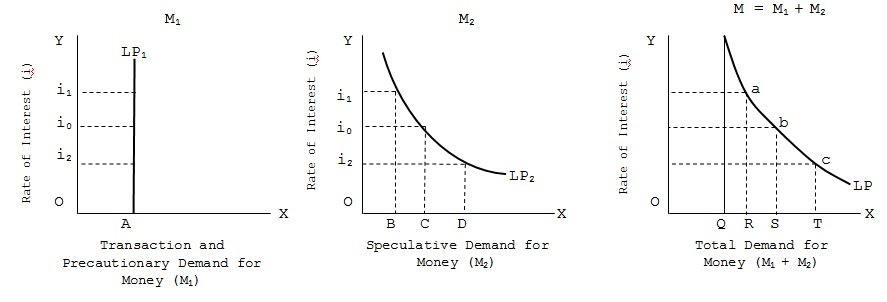Liquidity Preference Curve

# Liquidity Preference Curve Assignment Help

Home / Macroeconomics Assignment Help / Liquidity Preference Curve

# Liquidity Preference Curve

The transaction and precautionary demand for money (M1) is dependent upon income (Y) or GDP and, therefore, largely independent of the rate of interest. The speculative demand for money (M2) is dependent upon and varies inversely, with the rate of interest. Given the income level, the total demand for money M, i.e., (M1 + M2) will, thus, change inversely with changes in the market rate of interest, because M1 remains constant while M2 changes in the direction opposite to changes in the rate of interest. This can be shown below.In transaction and precautionary demand for money (M1) is shown along X-axis and rate of interest (i) is shown on Y-axis. Since, transaction and precautionary demand depend upon the GDP level and price level and is largely independent and unresponsive to interest rate changes, the liquidity preference curve with regard to these motives (LP1) is a parallel straight line at a distance of OA on the X-axis. This means, that money demand M1 is OA, whatever, be the rate of interest P0, P1 and P2.

In fig. Shows the speculative demand for money (M2), the liquidity preference curve LP2 showing the speculative demand for money is negatively sloped. It shows the lesser amount of money (OB) will be held for speculative purposes at high interest rate (i1) while a larger amount (OD) will be held at lower interest rate (i2).

In fig. is the total liquidity preference, i.e., total demand for money M1 + M2. The curve LP which shows the total demand for money (for all three purposes) is a horizontal summation of quantity of money demanded M1, and M2, with the same rate of interest measured on the Y-axis. Thus, in the amount of money balance held OQ is the same OA and has no relation to interest rate. But the amount OR shows OA, +OB, Similarly, OS = OA+OC and OT = OA+OD. Thus, the liquidity preference high interest rate and increase with a fall in the rate of interest. In other words, demand for money and rate of interest are negatively related.

It may be noted that the liquidity preference curve becomes flat and parallel to X-axis at low rates of interest below OP3. This is because the current rate of interest is very low and therefore the opportunity cost (viz., income foregone) of holding money as cash balance is negligible. Also there is no likelihood of interest rate falling further; rather, having reached the rock bottom, there is every chance of its going up in the near future. Very low rate of interest means that the security prices are at the highest possible level and are likely to crash very soon. Hence, there will be no buyers for the securities; people would like to hold all cash to buy securities only when their prices become low in future. Hence, this flat portion of the liquidity preference curve shows a high (almost infinite) demand for money.

### Submit Homework

Submit your homework for a free quote